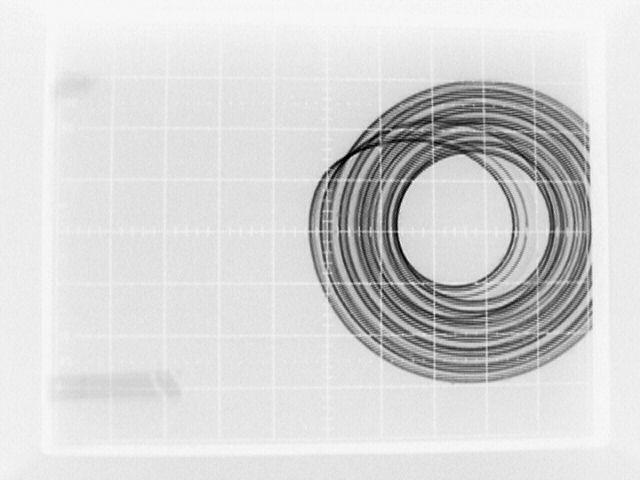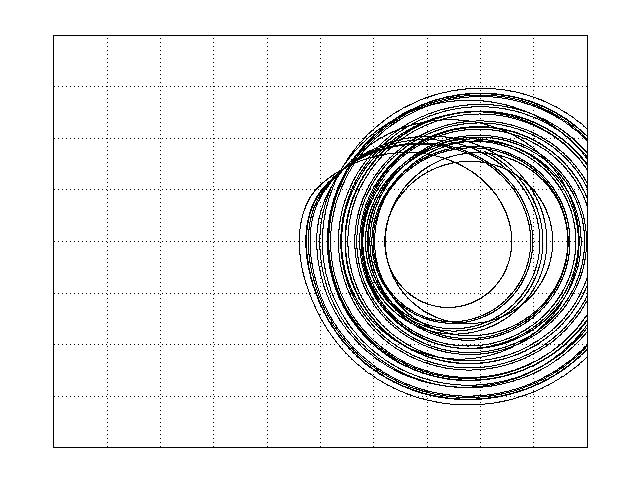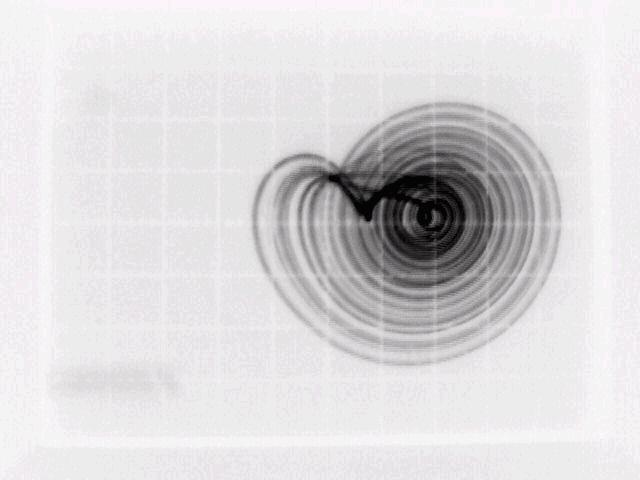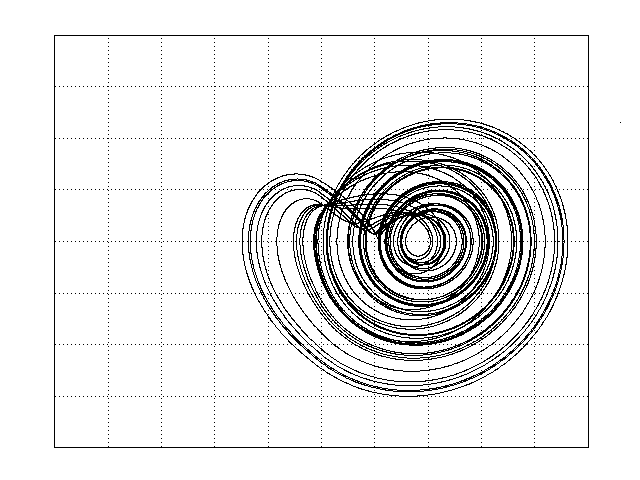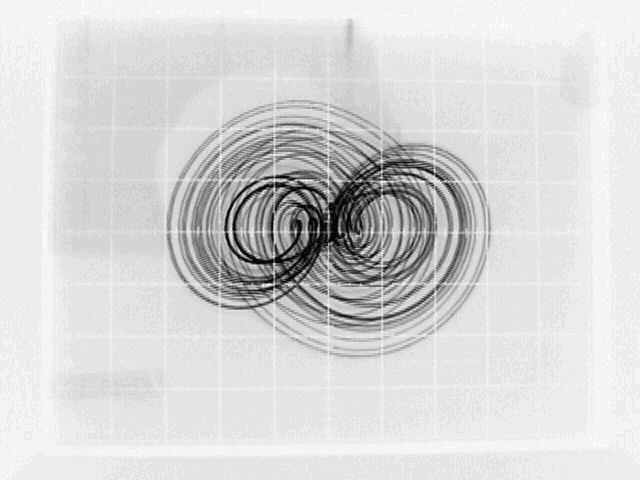# Simple Chaotic Systems and Circuits

J. C. Sprott
Department of Physics, University of Wisconsin, Madison, Wisconsin 53706
(Received 25 August 1999; accepted 29 November 1999)

### ABSTRACT

Many new chaotic systems with algebraically simple representations are described. These systems involve a single third-order autonomous ordinary differential equation (jerk equation) with various nonlinearities. Piecewise linear functions are emphasized to permit easy electronic implementation with diodes and operational amplifiers.  Several new simple and robust chaotic electrical circuits are described and evaluated.

Ref: J. C. Sprott, Am. J. Phys. 68, 758-763 (2000)

The complete paper is available in PDF format.

Fig. 1. Some mathematical operations that can be performed with operational amplifiers and ideal diodes.  In each case the inverting (-) input to the op amp is used, and the noninverting (+) input is grounded.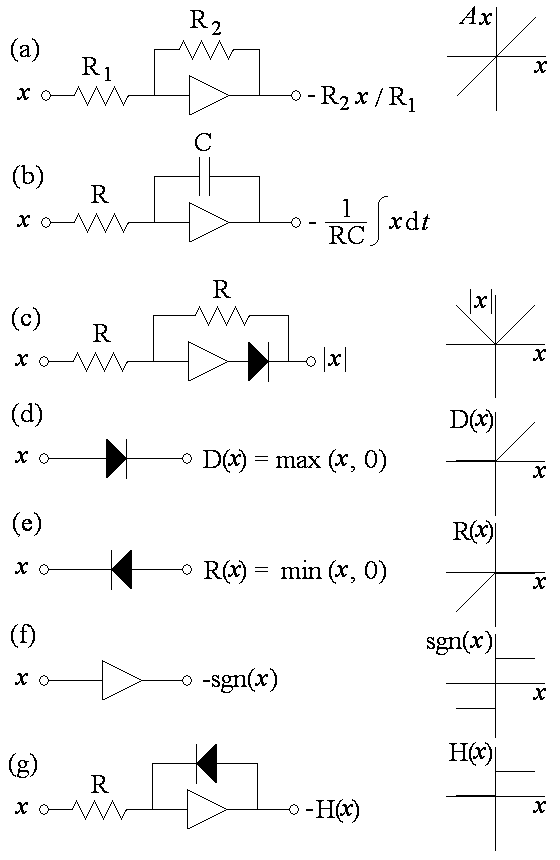Fig. 2. Chaotic circuit implementation of Eq. (5) using inverting op amps. The diodes are germanium, the battery is 1 V, the capacitors are 0.1 microfarads, and the resistors are 1 kilo-ohm except for the variable resistor, which should be adjustable from 1 to 2 kilo-ohms.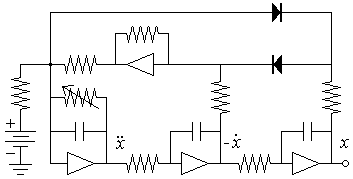Fig. 3. Chaotic circuit implementation of Eq. (7) using three op amps. Capacitances are in microfarads, and all resistors are 1 kilo-ohm. The resistor in series with the battery can be increased to prevent saturating the op amps.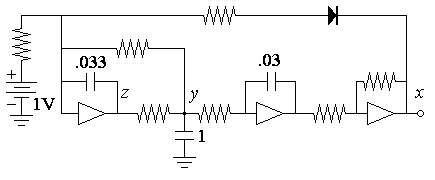Fig. 4. Chaotic circuit implementation of Eq. (8) using three op amps. Capacitances are in microfarads, and all resistors are 1 kilo-ohm.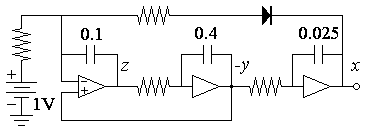Fig. 5. Chaotic circuit implementation of Eq. (9) with the plus sign. Capacitances are in microfarads, and all resistors are 1 kilo-ohm except for R, which should be adjusted to give a current of 1 mA when the upper op amp is saturated.Fig. 6. Attractors produced by Eq. (10) in the x-dx/dt plane. In the left-hand column are oscilloscope traces for a circuit like Fig. 5 but with an extra inversion, and in the right-hand column are the corresponding numerical solutions on the same scale.